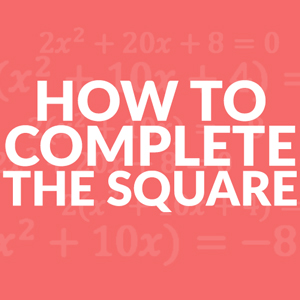##How to Complete the Square in Algebra

Completing the square, sometimes called x 2 x 2, is a method that is used in algebra to turn a quadratic equation from standard form, ax+ bx + c, into vertex form, a(x-h)+ k.

The vertex form is an easy way to solve, or find the zeros of quadratic equations. It also helps to find the vertex (h, k) which would be the maximum or minimum of the equation.

## Steps to Complete the Square

Here are the operations and x 2 x 2 steps to complete the square in algebra.

1. Start by factoring out the a
2. Move the c term to the other side of the equation.
3. Use the b term in order to find a new c term that makes a perfect square. This is done by first dividing the b term by 2 and squaring the quotient and add to both sides of the equation.
4. Find your h, the b term divided by two, for the perfect square.
5. Set equation to zero.

## Completing the Square Formula

For example, if a ball is thrown and it follows the path of the completing the square equation x+ 6x – 8 = 0.

The maximum height of the ball or when the ball it’s the ground would be answers that could be found when the equation is in vertex form. When you complete the square you can get the equation (x+3)– 17 = 0.

## Completing the Square Examples

The first example is going to be done with the equation from above since it has a coefficient of 1 so a = 1.

Step #1 – Move the c term to the other side of the equation using addition.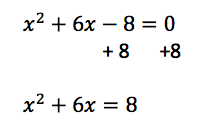Step #2 – Use the b term in order to find a new c term that makes a perfect square. This is done by first dividing the b term by 2 and squaring the quotient.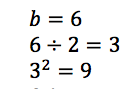This number gets added to both sides of the equation to maintain the balance of the equation. What you do to one side, you do to the other side.

Step #3 – Simplify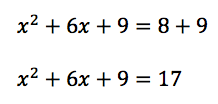Your new perfect square, the h, is the b term divided by two. This is due to the fact that you are splitting that term into two parts.

x2 + 3x + 3x + 9 = (x+3)2

Step #4 – Last step is to set the equation to zero by using subtraction 2x+ 20x + 8 = 0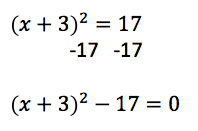## Solving General Quadratic Equations by Completing the Square

Now what about when a is not equal to zero?

Let’s start with the following equation: 2x+ 20x + 8 = 0

Step #1 – Start by factoring out the a term, divide each term by 2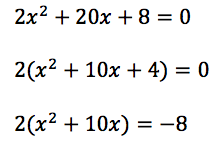Step #2 – Move the c term to the other side of the equation using subtraction.

*You are not subtracting 4, but subtracting since the a term was factored    out. Look back at the original equation, the c term is 8.

Step #3 – Find your new c term.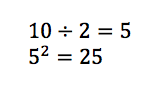When adding the new c term to both sides, keep in mind that once more you need to multiply it by the factored a term.

2(x+ 10x + 25) = -8 + 50

Simplify

2(x+ 10x + 25 ) = 42

Step #4 – Your new perfect square, the h, is the b term divided by two. This is due to the fact that you are splitting that term into two parts.

x2 + 5x + 5x + 9 = (x + 5)2

Step #5 – Set the equation to 0 by using subtraction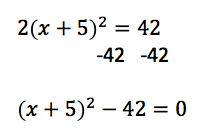## Why is Completing the Square Important?

By completing the square, a quadratic equation is in vertex form. It is easier to find the zeros of the equation in this form. That information is often The starting point or ending point for many equation. For example, when an object is touching the ground.

It is also easy to find the vertex of the equation. The vertex is either the maximum point or the minimum point of the equation. This is great for when you want to find the maximum profit or volume given certain restraints.